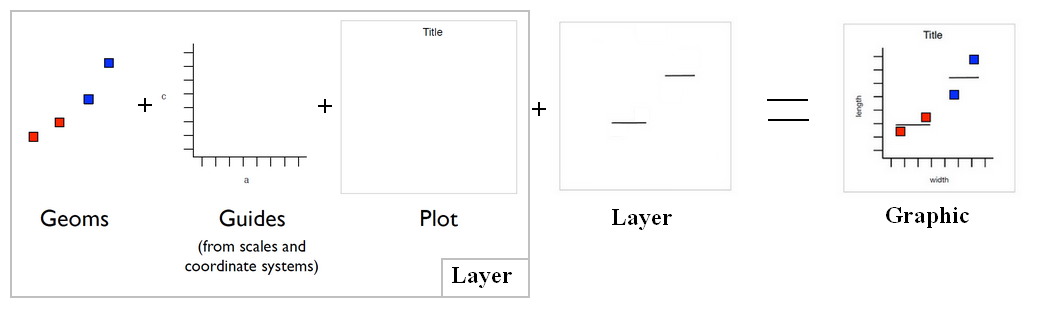# GGplot - Layer (data + mapping + geom + stat + position)

• data: The data frame (in a variable or a function, if null inherited)
• + mapping: Mapping data to geom properties (ie aesthetics)
• + geom : The geometric object to use display the data
• + stat: The statistical transformation to use on the data

Let op: geom, stat, position and annotate have also shortcut functions that creates layer. Example:

• geom_point() will create a layer for a point geom.
• stat_summary()

## Syntax

````layer(...)`
```

Discover MoreGgplot - Bars, rectangles with bases on x-axis (Geom_bar)

A bar geom makes the height of the bar proportional to: the number of cases in each group (default) or the sum of a column (using the weight aesthetic) The geom_bar layer is used to produce 1d area...Ggplot - Geom (geometric objects) - type of plot

Geom is a short for geometric objects that describe the type of plot produced. point for point (to create a scatterplot) line for a line ... The geom_... functions are shortcuts for a layer set...Ggplot - Smooth (Geom_Smooth|Stat_Smooth)

smoothing in Ggplot Smooth (Smoothed conditional means) is seen as a: stat - mean calculation and a geom - line or point geom_smoothstat_smooth() is a layer and an alias of geom_smoothgeom_smooth()....R - Ggplot

ggplot is a graphic library that follows the 0387245448grammar of graphics. charts are made of : layers that are made of: Data geometries: the graphical element...